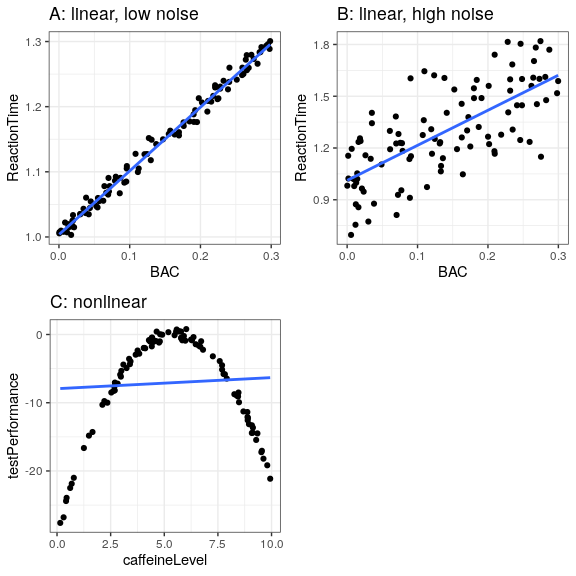# 8.4: What Makes a Model “Good”?

$$\newcommand{\vecs}{\overset { \rightharpoonup} {\mathbf{#1}} }$$ $$\newcommand{\vecd}{\overset{-\!-\!\rightharpoonup}{\vphantom{a}\smash {#1}}}$$$$\newcommand{\id}{\mathrm{id}}$$ $$\newcommand{\Span}{\mathrm{span}}$$ $$\newcommand{\kernel}{\mathrm{null}\,}$$ $$\newcommand{\range}{\mathrm{range}\,}$$ $$\newcommand{\RealPart}{\mathrm{Re}}$$ $$\newcommand{\ImaginaryPart}{\mathrm{Im}}$$ $$\newcommand{\Argument}{\mathrm{Arg}}$$ $$\newcommand{\norm}{\| #1 \|}$$ $$\newcommand{\inner}{\langle #1, #2 \rangle}$$ $$\newcommand{\Span}{\mathrm{span}}$$ $$\newcommand{\id}{\mathrm{id}}$$ $$\newcommand{\Span}{\mathrm{span}}$$ $$\newcommand{\kernel}{\mathrm{null}\,}$$ $$\newcommand{\range}{\mathrm{range}\,}$$ $$\newcommand{\RealPart}{\mathrm{Re}}$$ $$\newcommand{\ImaginaryPart}{\mathrm{Im}}$$ $$\newcommand{\Argument}{\mathrm{Arg}}$$ $$\newcommand{\norm}{\| #1 \|}$$ $$\newcommand{\inner}{\langle #1, #2 \rangle}$$ $$\newcommand{\Span}{\mathrm{span}}$$$$\newcommand{\AA}{\unicode[.8,0]{x212B}}$$

There are generally two different things that we want from our statistical model. First, we want it to describe our data well; that is, we want it to have the lowest possible error when modeling our data. Second, we want it to generalize well to new datasets; that is, we want its error to be as low as possible when we apply it to a new dataset. It turns out that these two features can often be in conflict.

To understand this, let’s think about where error comes from. First, it can occur if our model is wrong; for example, if we inaccurately said that height goes down with age instead of going up, then our error will be higher than it would be for the correct model. Similarly, if there is an important factor that is missing from our model, that will also increase our error (as it did when we left age out of the model for height). However, error can also occur even when the model is correct, due to random variation in the data, which we often refer to as “measurement error” or “noise”. Sometimes this really is due to error in our measurement – for example, when the measurements rely on a human, such as using a stopwatch to measure elapsed time in a footrace. In other cases, our measurement device is highly accurate (like a digital scale to measure body weight), but the thing being measured is affected by many different factors that cause it to be variable. If we knew all of these factors then we could build a more accurate model, but in reality that’s rarely possible.

Let’s use an example to show this. Rather than using real data, we will generate some data for the example using a computer simulation (about which we will have more to say in a few chapters). Let’s say that we want to understand the relationship between a person’s blood alcohol content (BAC) and their reaction time on a simulated driving test. We can generate some simulated data and plot the relationship (see Panel A of Figure 8.5).Figure 8.5: Simulated relationship between blood alcohol content and reaction time on a driving test, with best-fitting linear model represented by the line. A: linear relationship with low measurement error. B: linear relationship with higher measurement error. C: Nonlinear relationship with low measurement error and (incorrect) linear model

In this example, reaction time goes up systematically with blood alcohol content – the line shows the best fitting model, and we can see that there is very little error, which is evident in the fact that all of the points are very close to the line.

We could also imagine data that show the same linear relationship, but have much more error, as in Panel B of Figure 8.5. Here we see that there is still a systematic increase of reaction time with BAC, but it’s much more variable across individuals.

These were both examples where the linear model seems appropriate, and the error reflects noise in our measurement. The linear model specifies that the relationship between two variables follows a straight line. For example, in a linear model, change in BAC is always associated with a specific change in ReactionTime, regardless of the level of BAC.

On the other hand, there are other situations where the linear model is incorrect, and error will be increased because the model is not properly specified. Let’s say that we are interested in the relationship between caffeine intake and performance on a test. The relation between stimulants like caffeine and test performance is often nonlinear - that is, it doesn’t follow a straight line. This is because performance goes up with smaller amounts of caffeine (as the person becomes more alert), but then starts to decline with larger amounts (as the person becomes nervous and jittery). We can simulate data of this form, and then fit a linear model to the data (see Panel C of Figure 8.5). The blue line shows the straight line that bests fits these data; clearly, there is a high degree of error. Although there is a very lawful relation between test performance and caffeine intake, it follows a curve rather than a straight line. The linear model has high error because it’s the wrong model for these data.

This page titled 8.4: What Makes a Model “Good”? is shared under a not declared license and was authored, remixed, and/or curated by Russell A. Poldrack via source content that was edited to the style and standards of the LibreTexts platform; a detailed edit history is available upon request.